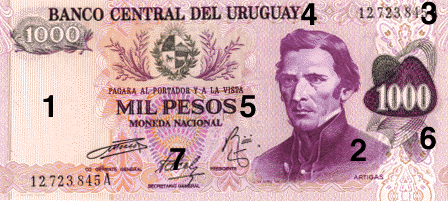###### Fancy Number Banknotes

________________________________________

Many collectors specialize in collecting banknotes with unusual or fancy serial numbers. Some banknotes with sought after numbers can be worth a lot more than those with random numbers.

A serial number that reads the same forwards and backwards is a radar note. For example, number 835538 shown on this note is a radar serial number because you can read the digits backward and it remains the same number.

Solid
If all the digits in the serial number are the same, it is called a solid note. Solid notes are extremely rare. Examples are 000000, 555555, 444444, and 888888. Serial number 000000 is usually reserved for specimen notes.
Eight (8) is considered the most lucky number for the Chinese people around the world. The word "eight" in Chinese sounds similar to the word meaning "prosperity". So notes with solid number 888888 are highly in demand. Four (4), on the other hand, is a number to avoid because it sounds similar to the word meaning "death".

Low Serial Number
The lower the serial number, the more valuable is the note. A serial number of 000001 is very hard to get.

Binary
A note with a serial number containing just two unique digits is a binary note. An example is number 252255.

Doubles
When a serial number contains pairs of numbers, it's a doubles note. Examples of doubles are 446600 and 228855.

Repeater
Serial numbers with a sequence of numbers that repeats itself is a repeater. Serial number 535353 is a repeater.

Consecutives
Consecutive notes are two or more different notes with consecutive serial numbers. You have two consecutive notes if one has a serial number 384655 and the other 384656.

Bookends
A bookend note has a serial number having the same sequence of digits at both ends. An example is serial number 639563. "63" is the sequence of digits appearing at both the beginning and end of the serial number.